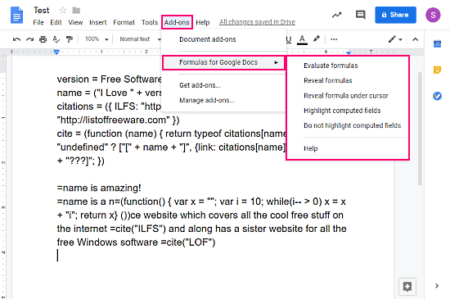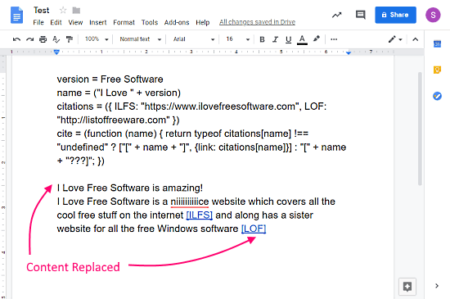Editor Ratings:
User Ratings:
[Total: 0 Average: 0]

Formulas in spreadsheets are very popular which come handy for calculations calling values, etc. Using formulas, you can increase your production and also save time. Formulas and variables can also be used to create dynamic documents which can be easily edited by changing the variables.

In this article, I will cover a method to use Formulas and Variables in Google DocsFormulas for Google Docs is a free and open source add-on for Google Docs that lets you define variables in the document and insert computations to produce rich text. With computations (formulas), you can create temporary placeholders in the documents which can be easily replaced by changing the variables. These formulas are not like the spreadsheet formulas and that’s because the purpose of using the formulas in the Google Docs is totally different from the spreadsheets.

## How to Use Formulas, Variables in Google Docs to Replace ContentUnlike spreadsheets, you won’t get any formula bar here. Instead, you have to do all the work in the document itself. First, you have to define the variables at the top of the document. Then, you can start writing your document and use the formulas wherever needed. The syntax of the formulas and variable is derived from JavaScript; you can find a complete syntax guide for this add-on here.• Evaluate Formulas: This option evaluates all the formulas and replaces them with appropriate content.
• Reveal Formulas: This option is opposite of the above; it reveals all the formulas used in the documents.
• Reveal Formula under the cursor: This option reveals the formulas from the selected text only.
• Highlight Computed Fields: This option highlights the content added by the formulas.
• Do not Highlight Computed Fields: This option is for reverting the content highlight.You can write the formulas throughout the entire documents and then replace them with the content with Evaluate Formulas option. For example, in the attachment above, first I defined names and citations as variables and then used formulas to call those values. As this add-on is based on JavaScript, you can use loops and other functions as well.